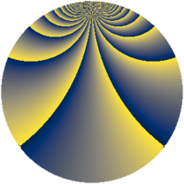# Properties

 Label 1470.2.bjLevel $1470$ Weight $2$ Character orbit 1470.bj Rep. character $\chi_{1470}(113,\cdot)$ Character field $\Q(\zeta_{28})$ Dimension $1344$ Sturm bound $672$

# Related objects

## Defining parameters

 Level: $$N$$ $$=$$ $$1470 = 2 \cdot 3 \cdot 5 \cdot 7^{2}$$ Weight: $$k$$ $$=$$ $$2$$ Character orbit: $$[\chi]$$ $$=$$ 1470.bj (of order $$28$$ and degree $$12$$) Character conductor: $$\operatorname{cond}(\chi)$$ $$=$$ $$735$$ Character field: $$\Q(\zeta_{28})$$ Sturm bound: $$672$$

## Dimensions

The following table gives the dimensions of various subspaces of $$M_{2}(1470, [\chi])$$.

Total New Old
Modular forms 4128 1344 2784
Cusp forms 3936 1344 2592
Eisenstein series 192 0 192

## Trace form

 $$1344q - 20q^{6} + 4q^{7} + O(q^{10})$$ $$1344q - 20q^{6} + 4q^{7} - 8q^{10} - 24q^{15} + 224q^{16} + 20q^{22} + 4q^{28} + 24q^{30} + 32q^{31} + 40q^{33} + 20q^{36} + 108q^{42} - 64q^{43} - 56q^{45} + 120q^{46} + 8q^{55} + 92q^{57} - 140q^{58} + 160q^{61} - 4q^{63} + 32q^{67} - 40q^{70} + 88q^{73} - 4q^{75} - 32q^{76} - 20q^{81} - 32q^{82} + 48q^{85} + 40q^{87} - 8q^{88} + 28q^{90} + 176q^{91} + 260q^{93} - 8q^{96} - 40q^{97} + O(q^{100})$$

## Decomposition of $$S_{2}^{\mathrm{new}}(1470, [\chi])$$ into newform subspaces

The newforms in this space have not yet been added to the LMFDB.

## Decomposition of $$S_{2}^{\mathrm{old}}(1470, [\chi])$$ into lower level spaces

$$S_{2}^{\mathrm{old}}(1470, [\chi]) \cong$$ $$S_{2}^{\mathrm{new}}(735, [\chi])$$$$^{\oplus 2}$$